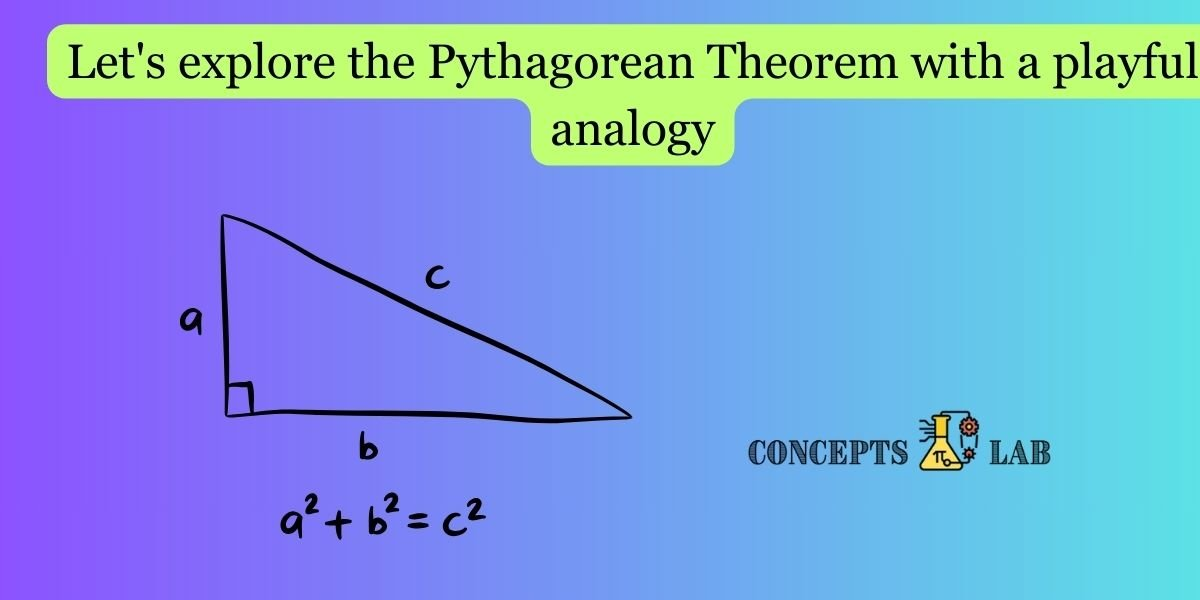Let’s explore the Pythagorean Theorem with a playful analogy using an enchanted garden and a friendly gnome. I’ll include a simple diagram to illustrate the concept.

Imagine you’re in an enchanted garden where paths are laid out like magic tiles, and you have a gnome friend named Pytho who loves to play with triangles.

Pythagorean Theorem: The Magical Garden Rule: Pytho tells you about his favorite magical rule called the Pythagorean Theorem, which says, “In a right-angled triangle, the square of the hypotenuse (the longest side) is equal to the sum of the squares of the other two sides.”

Diagram:

Here’s a simple diagram of a right-angled triangle in the enchanted garden:

``````           |
|\
| \
A     |  \  H
|   \
|____\
B
``````

In this triangle:

• Side AA is like the magical path going north.
• Side BB is like the magical path going east.
• Side HH (the hypotenuse) is the path going northeast, forming the right angle.

The Garden Squares: Along each path, there are garden squares, like magic tiles. Pytho explains that these squares love to dance when triangles are around.

The Hypotenuse Dance: Pytho points to the hypotenuse (path HH) and says, “Let’s count the magic tiles on this path and square the number.”

The Other Paths: He then points to the other two paths (paths AA and BB) and says, “Now, let’s count the magic tiles on each of these paths and square the numbers.”

Magical Connection: Pytho whispers, “Now, if we add the squared numbers of paths AA and BB, it will be exactly the same as the squared number of path HH!”

Magical Formula: Pytho reveals the magical formula: H^2 = A^2 + B^2H2=A2+B2. It’s like a spell that connects the lengths of the paths in a right-angled triangle.

Magical Dance of Tiles: To demonstrate, Pytho starts a magical dance. He counts the tiles on paths AA and BB, squares the numbers, adds them together, and voila! The total matches the number of tiles on the hypotenuse path HH.

Playful Experiment: Pytho encourages you to create your own right-angled triangles in the enchanted garden, count the magic tiles, and see if the Pythagorean Theorem holds true. It’s like creating your own magical dance of garden squares!

So, children, whenever you’re in a garden or see right-angled triangles, remember Pytho and the magical connection of the Pythagorean Theorem. It’s a playful way to understand the relationship between the sides of these special triangles!